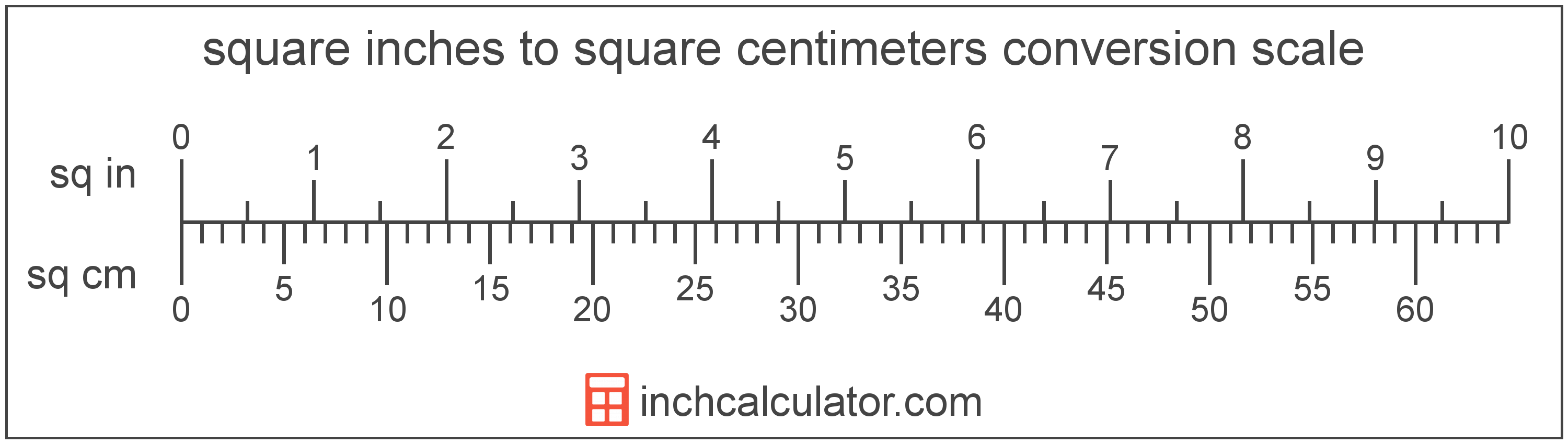# Square Centimeters to Square Inches Conversion

Enter the area in square centimeters below to get the value converted to square inches.

Results in Square Inches:1 sq cm = 0.155 sq in

## How to Convert Square Centimeters to Square InchesTo convert a square centimeter measurement to a square inch measurement, multiply the area by the conversion ratio.

Since one square centimeter is equal to 0.155 square inches, you can use this simple formula to convert:

square inches = square centimeters × 0.155

The area in square inches is equal to the square centimeters multiplied by 0.155.

For example, here's how to convert 5 square centimeters to square inches using the formula above.
5 sq cm = (5 × 0.155) = 0.775002 sq in

## Square Centimeters

One square centimeter is equal to the area of a square with 1 centimeter long sides.

The square centimeter, or square centimetre, is a multiple of the square meter, which is the SI derived unit for area. In the metric system, "centi" is the prefix for 10-2. A square centimeter is sometimes also referred to as a square cm. Square centimeters can be abbreviated as sq cm, and are also sometimes abbreviated as cm². For example, 1 square centimeter can be written as 1 sq cm or 1 cm².

## Square Inches

One square inch is equal to the area of a square with sides that are 1 inch in length.

The square inch is a US customary and imperial unit of area. A square inch is sometimes also referred to as a square in. Square inches can be abbreviated as sq in, and are also sometimes abbreviated as in². For example, 1 square inch can be written as 1 sq in or 1 in².

## Square Centimeter to Square Inch Conversion Table

Square centimeter measurements converted to square inches
Square Centimeters Square Inches
1 sq cm 0.155 sq in
2 sq cm 0.310001 sq in
3 sq cm 0.465001 sq in
4 sq cm 0.620001 sq in
5 sq cm 0.775002 sq in
6 sq cm 0.930002 sq in
7 sq cm 1.085 sq in
8 sq cm 1.24 sq in
9 sq cm 1.395 sq in
10 sq cm 1.55 sq in
11 sq cm 1.705 sq in
12 sq cm 1.86 sq in
13 sq cm 2.015 sq in
14 sq cm 2.17 sq in
15 sq cm 2.325 sq in
16 sq cm 2.48 sq in
17 sq cm 2.635 sq in
18 sq cm 2.79 sq in
19 sq cm 2.945 sq in
20 sq cm 3.1 sq in
21 sq cm 3.255 sq in
22 sq cm 3.41 sq in
23 sq cm 3.565 sq in
24 sq cm 3.72 sq in
25 sq cm 3.875 sq in
26 sq cm 4.03 sq in
27 sq cm 4.185 sq in
28 sq cm 4.34 sq in
29 sq cm 4.495 sq in
30 sq cm 4.65 sq in
31 sq cm 4.805 sq in
32 sq cm 4.96 sq in
33 sq cm 5.115 sq in
34 sq cm 5.27 sq in
35 sq cm 5.425 sq in
36 sq cm 5.58 sq in
37 sq cm 5.735 sq in
38 sq cm 5.89 sq in
39 sq cm 6.045 sq in
40 sq cm 6.2 sq in